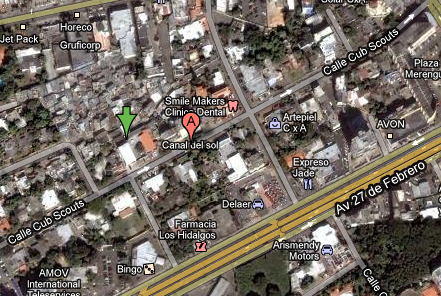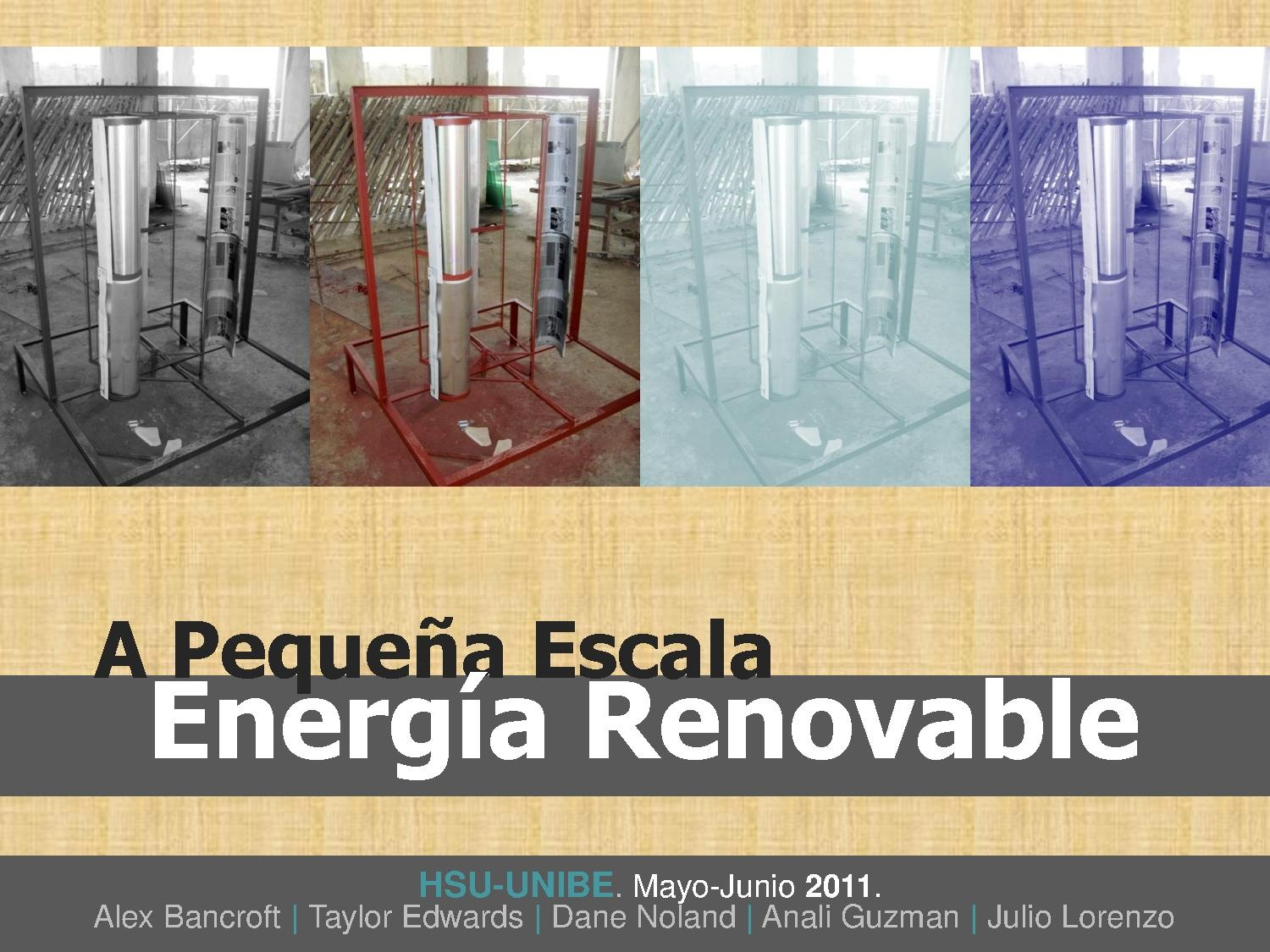# La Yuca small scale renewable energy 2011

Jump to navigation Jump to search

## Objective Statement

The objective of this Appropriate Technology project was to construct a small scale renewable energy system. This system was designed to serve as an educational tool, reduce fossil fuel use, along with having an acceptable pay-back time.

## Background

In the summer of 2011 the Humboldt State University Dominicana Program worked in collaboration with Universidad Iberoamericana (UNIBE), Colectivo RevARK, and the community of La Yuca, Santo Domingo. Together, they worked on exploring solutions to the energy demands of La Yuca. A prototype was designed and was scaled for construction. The finished product consists of two components (figure 1). These components include small scale vertical axis wind turbine and a 10 watt solar panel.

## Location

La Yuca del Naco, Santo Domingo, Dominican Republic## Criteria

Table 1: Criteria

Criteria Weight Description
Safety 10 Safety is the ability of the design to prevent injury of any kind. The small scale renewable energy system must not be unsafe.
Effectiveness 10 The effectiveness of the small scale renewable energy system is the extent to which the system transfers energy, can be used as an educational tool, and the system's ability to be marketed locally.
Durability 10 Durability is the ability of the system to sustain a functioning state.
Aesthetics 5 Aesthetics is the system's level of visual appeal.
Turbine (construction labor) $20,000 (donated) 1 20,000 533.33 free free Black Metal Pipe 635 1 635 16.93 635 16.93 Angular Iron 2,435.47 1 2,435.47 64.95 2,435.47 64.95 Total =$35,411.47 $944.31$12,225.47 $326.01 # Timeline # Results ## Payback Time The payback time of the system has been divided into two parts. The payback time has been calculated separately for the wind turbine and the solar panel. These calculations were made assuming that the user uses all of the energy that the system is able to output. Maintenance costs and change in energy prices are not factored into these calculations. ### Wind Turbine The wind turbine's payback time was calculated using an estimated energy output of $1 kWh/10 days$ or $0.1 kWh/day$. Also, the payback period was calculated excluding any labor costs as these services were donated. The price of energy that was used to calculate the payback period was $0.136/kWh$. The payback period of the wind turbine is approximately 59.63 years (Figure 7). Two equations were used to calculated the simple payback time:: $A_sv = E_sv*c$ $A_sv = AnnualSavings$ $E_sv = Energy Savings/year$ $c = EnergyCost/unit$ $P = I/A_sv$ $P = Payback Period (yrs)$ $I = System Initial Cost$ $A_sv = Annual Energy Savings$ ### Solar Panel The payback period of the solar panel was calculated to be 14.9 years (Figure 8). An average of 5.07 hours of full sun per day was used in the calculations. The data was also used to show the relationship between monthly full-sun hours and monthly savings (Figure 9). The cost of energy used to calculate the panel's payback period was $0.136/kWh$. The initial cost of the solar panel was$30 USD. Several equations were used to calculate the panel's simple payback period:

$Esv=Ppv*S*n*365days/yr$
$E_sv = Energy Savings/year$
$P_pv = Rated Power Of Panel$
$S = FullSunHours/day$
$n = Efficiency$
$A_{sv} = Esv \times c$
$Asv = AnnualSavings$
$Esv = Energy Savings/year$
$c = EnergyCost/unit$
$P = \frac{I}{A_{sv}}$
$P = Payback Period (yrs)$
$I = System Initial Cost$
$A_sv = Annual Energy Savings$

## Wind Turbine Efficiency

The wind turbine's efficiency was calculated using an estimated output of $0.10kWh/day$, an air density of $1.225 kg/m^3$, a yearly average wind velocity of $6.27m/s$, and the wind turbine's wind swept area of $1.115m^2$. It is important to note that this efficiency calculation produces an instantaneous efficiency. In this case the instantaneous efficiency was calculated using an average wind velocity. The calculated efficiency of the wind turbine will vary depending upon the instantaneous conditions of the system such as velocity and air density. The instantaneous efficiency was calculated to be 4.53%.

The mathematical equations used to calculate this efficiency are shown below:

$P = p*A*V^3$
$P = PowerPontential$
$p = air density$
$A = WindSweptArea$
$V = velocity of wind$
$P$1$*n=P$2
$P$1$= PowerPotential$
$P$2$= PowerOutput$
$n = efficiency$

## Next Step

The next step is to utilize the knowledge gained through this design process by applying it to future projects. By applying this gained experience to future projects, more effective systems may be produced. In this case, the system's effectiveness was measured using payback time. Many components of the design can be improved upon to reduce the turbine's estimated payback time of 59.63 years.

Reducing the initial construction costs of the turbine will lower the payback time significantly. Reducing these costs can be done in various ways. For example, the turbine's frame could have been constructed using mostly rebar instead of angle iron. Furthermore, the solar charge controller could have been homemade instead of store bought, which could save about \$24.

Another area of significant improvement could be the systems gearing. Special care should be put into the configuring and placement of the gears so that they may operate as smoothly as possible. Less friction the gearing system would allow more of the kinetic energy of the wind to be transferred into electrical energy. Also, selecting a sufficient gear ratio is crucial in optimizing energy output. Both of these improvements could greatly improve the efficiency, and energy production of the wind turbine system.

## Helpful FilesPower Point Presentation on PDF